# 3-room apartment Les 2 Alpes - Page 5

Viewing mode
129 results
• Slopes access :
• ski-in (-50 m)
• Rooms :
• 3 rooms
• Total surface (sq.m) :
• 38 sq.m
•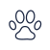•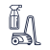• Garage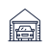•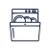•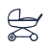• Slopes access :
• 50 -300m to the slopes
• Rooms :
• 3 rooms
• Total surface (sq.m) :
• 65 sq.m
••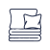••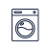•• Slopes access :
• ski-in (-50 m)
• Rooms :
• 3 rooms
• Total surface (sq.m) :
• 47 sq.m
•••• Slopes access :
• ski-in (-50 m)
• Rooms :
• 3 rooms
• Total surface (sq.m) :
• 60 sq.m
•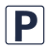•••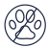• Slopes access :
• 50 -300m to the slopes
• Rooms :
• 3 rooms
• Total surface (sq.m) :
• 80 sq.m
•••••• Slopes access :
• 50 -300m to the slopes
• Rooms :
• 3 rooms
• Total surface (sq.m) :
• 44 sq.m
••••• Slopes access :
• ski-in (-50 m)
• Rooms :
• 3 rooms
• Total surface (sq.m) :
• 55 sq.m
•••••• Slopes access :
• ski-in (-50 m)
• Rooms :
• 3 rooms
• Total surface (sq.m) :
• 60 sq.m
• Slopes access :
• 50 -300m to the slopes
• Rooms :
• 3 rooms
• Total surface (sq.m) :
• 45 sq.m
••••• Slopes access :
• ski-in (-50 m)
• Rooms :
• 2 rooms + cabin(s)
• 3 rooms
• Total surface (sq.m) :
• 53-68 sq.m
•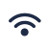•••••••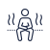•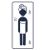• Slopes access :
• ski-in (-50 m)
• Rooms :
• 3 rooms
• Total surface (sq.m) :
• 42 sq.m
••••• Slopes access :
• 50 -300m to the slopes
• Rooms :
• 3 rooms
• Total surface (sq.m) :
• 59 sq.m
•••• Rooms :
• 3 rooms
• Total surface (sq.m) :
• 110 sq.m
••••• Slopes access :
• 300 - 500m to the slopes
• Rooms :
• 3 rooms
• Total surface (sq.m) :
• 43 sq.m
••••••• Slopes access :
• 50 -300m to the slopes
• Rooms :
• 3 rooms
• Total surface (sq.m) :
• 48 sq.m
••••• Slopes access :
• 50 -300m to the slopes
• Rooms :
• 3 rooms
• Total surface (sq.m) :
• 72 sq.m
••••••• Slopes access :
• 300 - 500m to the slopes
• Rooms :
• 3 rooms
• Total surface (sq.m) :
• 48 sq.m
••••••• Slopes access :
• ski-in (-50 m)
• Rooms :
• 3 rooms
• Total surface (sq.m) :
• 35 sq.m
•••••• Slopes access :
• 50 -300m to the slopes
• Rooms :
• 3 rooms
• Total surface (sq.m) :
• 44 sq.m
••••• Slopes access :
• 50 -300m to the slopes
• Rooms :
• 3 rooms
• 3 rooms + cabin(s)
• Total surface (sq.m) :
• 42 sq.m
••••